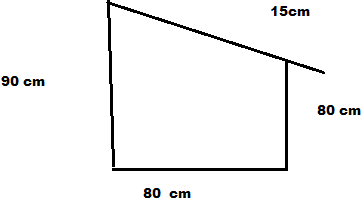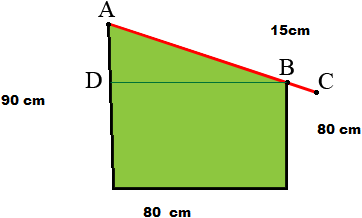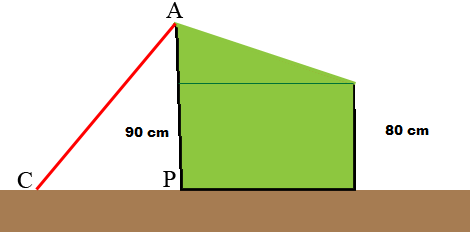SEARCH HOMEMath Central Quandaries & QueriesHi! I have another problem. The diagram shows a side view of a box which is used to store small logs of wood for burning in a fire place. The slopping lid has an overhand of 15cm. a) Calculate the total length of the slopping lid to the nearest cm. b) When the lid is open above ground will the end of the lid be? P.S. Please see attached. SophiaHere is the diagram Sophia sentHi Sophia,

I redrew the diagram because I am not sure which measurement is 15 cm. I also added some labels and a line segment.I am going to assume that the distance from B to C is 15 cm.

Triangle ADB is a right triangle and you know the lengths of AD and DB so Pythagoras' Theorem will tell you the length of AB. Add 15 cm to this to obtain the length of the lid, AC.

Next I put the box on the ground and opened the lid.I think part b) of the problem is asking for the distance from C to P. This looks like another job for Pythagoras' Theorem.

PennyMath Central is supported by the University of Regina and The Pacific Institute for the Mathematical Sciences.